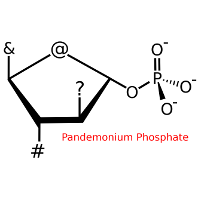## Free STEM Artwork for the Holidays!

 Astronomical Symbols Pythagorean Theorem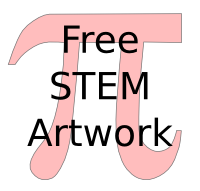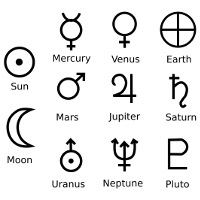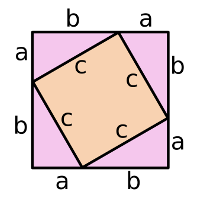Vacuum Tubes Four Classical Elements Hexadecimal Pi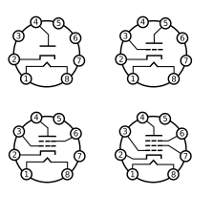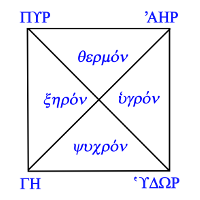Pythagorean Theorem Latin Long Division Maxwell's Thermodynamic Equations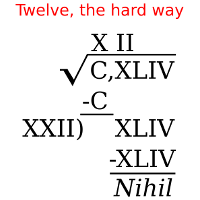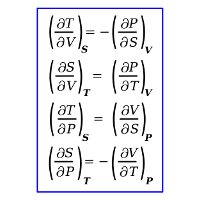Maxwell's Electromagnetic Equations SI Unit Prefixes Feral Network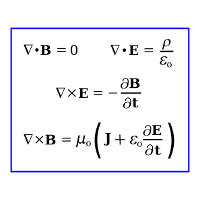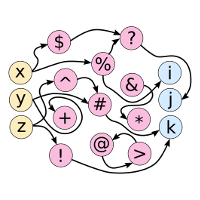Coffee Algorithm (F) Coffee Algorithm (C) Decimal Pi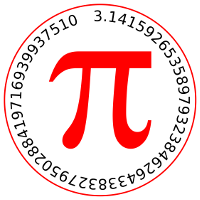Logic Equation Pandemonium Phosphate